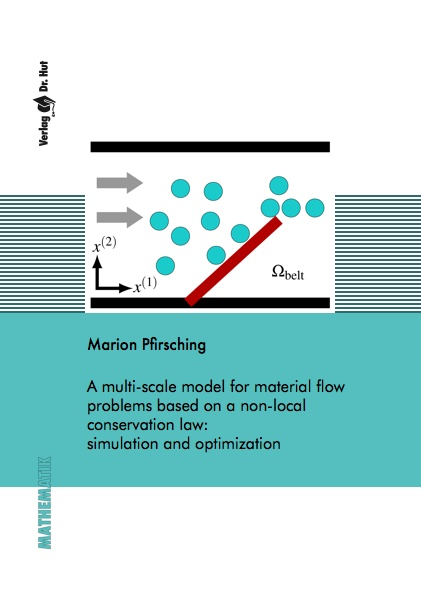Datenbestand vom 25. Juli 2020aktualisiert am 25. Juli 2020

# ISBN 978384393609539,00 € inkl. MwSt, zzgl. Versand

978-3-8439-3609-5, Reihe Mathematik

Marion Pfirsching
A multi-scale model for material flow problems based on a non-local conservation law: simulation and optimization

154 Seiten, Dissertation Universität Mannheim (2018), Softcover, A5

## Zusammenfassung / Abstract

This work examines a multi-scale model to simulate material flow on conveyor belts. Its aim is to describe the transport of circular objects. The model consists of a microscopic level, where each individual object is considered, and a macroscopic level, where the behavior of the entity of all objects over time is simulated. The microscopic level is described by a system of ordinary differential equations, whereas the macroscopic level is modeled by a partial differential equation, more exactly by a hyperbolic conservation law in two spatial dimensions. This second model is advantageous whenever the number of transported goods is too large to compute a trajectory for each object.

In this thesis, a switching method to transform the conguration of one level into the other at a-priori fixed points in time is derived. The macroscopic to microscopic level is the challenging direction since exact information of the object placement is missing. It is solved by a randomized placement according to the mass together with a nonlinear least squares problem. This switching method is also applicable to more general problems. Exemplary, it is applied to a model describing pedestrian flow.

The second focus is the optimization of the object placement on the belt. For each level, an optimal control problem is set up, once restricted by the microscopic model equations and once by the macroscopic ones. The method of adjoint optimization is appropriate here and is used to solve both systems. As the optimization of the macroscopic conservation law provides the best results, they are interpreted microscopically to answer the original problem.

Finally, a conservation law in one spatial dimension, describing material flow on a one-dimensional conveyor belt this time, is set up and applied to a network. Junction conditions for the nodes of the network are stated and the solution is derived analytically. Also, a numerical solution method is stated, whose results match very well the analytical solution.